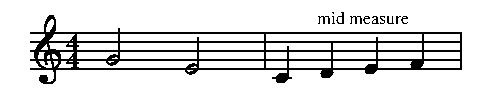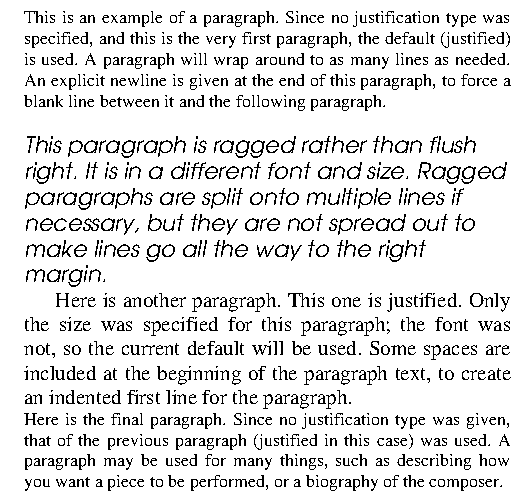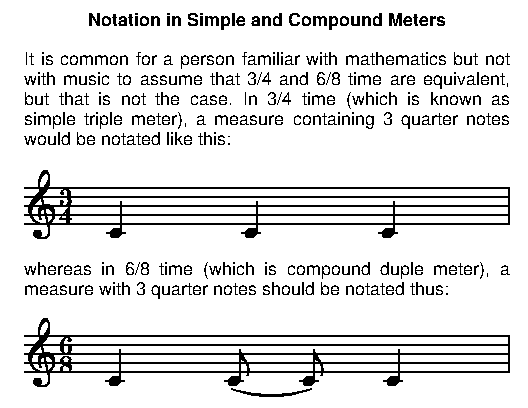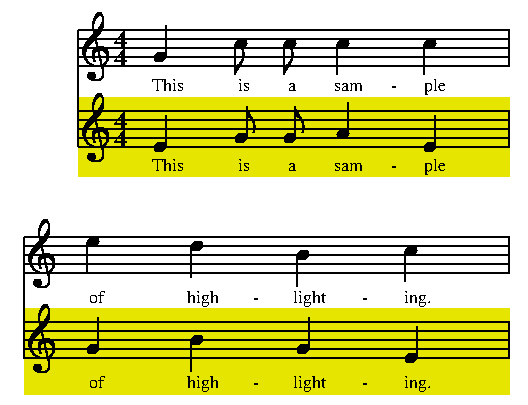## Lines and curves

The "line" statement is used for drawing lines. Its format is:

```linetype line (X1, Y1) to (X2, Y2)
```

The linetype can be "wide," "medium," "wavy," "dotted," "dashed," or it can be omitted, which means narrow. The wavy line could be used for glissandos. It could also be used for manually drawing rolls and trills, although it should rarely, if ever, be necessary to resort to this, since the roll and mussym statements will usually suffice and are much easier to use. Narrow, dashed, or dotted lines would typically be used for voice crossings. The medium line is about twice as wide as a narrow line, and a wide line is about twice as wide as a medium line.

The x and y coordinates are specified as was described in the section on "Location tags."

```score
vscheme=2f
staff2 clef=bass

music
1 1: c+ =c;e =e;g;c+;
1 2: e =_e;g =_g;c =_c;s;
2 1: 2.s;4g =_gg;
2 2: 1ce;
line (c.e + 2, c.y) to (e.w - 2, e.y)
line (_e.e + 2, _e.y) to (_g.w - 2, _g.y)
dashed line (_c.e + 1, _c.y) to (_gg.w - 1, _gg.y)
bar
```It is possible to print a text string by the line by adding

```with fontfamily font (size) "string"
```

at the end of the "line" statement. Only the keyword "with" and the text string itself are required; the font and size information is optional. As usual, the fontfamily, font, and size parameter values are used to get values if the optional items are omitted. The most common usage for printing a string with a line is probably for glissandos.
```1: 2d =n; g+ =m;
wavy line (n.e + 1.5, n.y) to (m.w - 1.5, m.y) with ital (9) "gliss."
bar
```Arbitrary curves can be drawn using the "curve" statement:

```linetype curve (X1,Y1) to (X2,Y2) to (X3,Y3) ...
```

When using this form of the "curve" statement, at least three coordinates must be specified; more are permitted. The linetype can be "medium," "wide," "dashed," or "dotted," or omitted. A curve will be drawn through the specified points in the specified order.
```score
staffs=2

staff 2
clef=bass

music
1: 4.s;[=c] 8e; [=d] g; [=e] e;4s;
2: [=a] 8c;g; [=b] c+;4.s; [=f] 8c+; [=g] c;
medium curve (a.x, a.n+1) to (b.x, b.n+15) to (c.x, c.n+4) \
to (d.x, d.n+3) to (e.x, e.n+3) \
to (f.x, f.n+11) to (g.x, g.n+1)
bar
```An alternative way to specify a curve is to only specify the two endpoints, along one or more "bulge" values, given in stepsizes. Suppose a single bulge value n is given. To find the midpoint of the resulting curve, imagine a line is drawn between the two endpoints, then from the midpoint of that line, move perpendicular to the line for n stepsizes. A positive n will cause the curve to bulge to the left as you move from the start point to the end point, while a negative n will make it bulge to the right. If two bulge values are given, bulges are calculated from the 1/3 and 2/3 point of the line between the endpoints; with three points, at 1/4, 1/2, and 3/4, and so forth. Multiple bulge values are separated by commas.

```1: c =_c1; f; f; d =_d1;
medium curve (_c1.x, _c1.y + 8) to (_d1.x, _d1.y + 10) bulge 3
bar

1: g =_g1; e+; d+; a =_a1;
dotted curve (_g1.x, _g1.y - 2) to (_a1.x, _a1.y - 2) bulge -4.3
bar

1: g =_g2; a; d+; c+ =_c2;
curve  (_g2.x, _g2.y - 2) to (_c2.x, _c2.y + 2) bulge -2.5, 4
bar
```